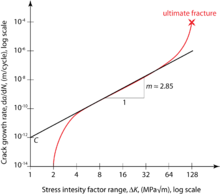# Paris' law

Paris' lawSchematic plot of the typical relationship between the crack growth rate and the range of the stress intensity factor. In practice, the Paris law is calibrated to model the linear interval around the center.

Paris' law (also known as the Paris-Erdogan law) relates the stress intensity factor range to sub-critical crack growth under a fatigue stress regime. As such, it is the most popular fatigue crack growth model used in materials science and fracture mechanics. The basic formula reads$\frac{{\rm d}a}{{\rm d}N} = C \Delta K^m$,

where a is the crack length and N is the number of load cycles. Thus, the term on the left side, known as the crack growth rate, denotes the infinitesimal crack length growth per increasing number of load cycles. On the right hand side, C and m are material constants, and ΔK is the range of the stress intensity factor, i.e., the difference between the stress intensity factor at maximum and minimum loading

ΔK = KmaxKmin,

where Kmax is the maximum stress intensity factor and Kmin is the minimum stress intensity factor.

## History and use

The formula was introduced by P.C. Paris in 1961. Being a power law relationship between the crack growth rate during cyclic loading and the range of the stress intensity factor, the Paris law can be visualized as a linear graph on a log-log plot, where the x-axis is denoted by the range of the stress intensity factor and the y-axis is denoted by the crack growth rate.

Paris' law can be used to quantify the residual life (in terms of load cycles) of a specimen given a particular crack size. Defining the crack intensity factor as$K=\sigma Y \sqrt{\pi a}$,

where σ is a uniform tensile stress perpendicular to the crack plane and Y is a dimensionless parameter that depends on the geometry, the range of the stress intensity factor follows as$\Delta K=\Delta\sigma Y \sqrt{\pi a}$,

where Δσ is the range of cyclic stress amplitude. Y takes the value 1 for a center crack in an infinite sheet. The remaining cycles can be found by substituting this equation in the Paris law$\frac{{\rm d}a}{{\rm d}N} = C \Delta K^m =C(\Delta\sigma Y \sqrt{\pi a})^m$.

For relatively short cracks, Y can be assumed as independent of a and the differential equation can be solved via separation of variables$\int^{N_f}_0 {\rm d}N = \int^{a_c}_{a_i}\frac{{\rm d}a}{C(\Delta\sigma Y \sqrt{\pi a})^m } =\frac{1}{C(\Delta\sigma Y \sqrt{\pi})^m }\int^{a_c}_{a_i} a^{-\frac{m}{2}}\;{\rm d}a$

and subsequent integration$N_f=\frac{2\;(a_c^{\frac{2-m}{2}}-a_i^{\frac{2-m}{2}})}{(2-m)\;C(\Delta\sigma Y \sqrt{\pi})^m}$,

where Nf is the remaining number of cycles to fracture, ac is the critical crack length at which instantaneous fracture will occur, and ai is the initial crack length at which fatigue crack growth starts for the given stress range Δσ. If Y strongly depends on a, numerical methods might be required to find reasonable solutions.

For the application to adhesive joints in composites, it is more useful to express the Paris Law in terms of fracture energy rather than stress intensity factors.

Wikimedia Foundation. 2010.

Нужен реферат?

### Look at other dictionaries:

• Law, John — (baptized April 21, 1671, Edinburgh, Scot. died March 21, 1729, Venice) Scottish monetary reformer. In 1705 he published the banking reform plan Money and Trade Considered, in which, unlike other mercantilists, he proposed a central bank as an… …   Universalium

• Law & Order (franchise) — Law Order franchise Law Order title card Creator Dick Wolf Original work Law Order …   Wikipedia

• Law enforcement in the United Kingdom — Crime in the UK · Terrorism in the UK Topics …   Wikipedia

• PARIS — PARIS, capital of france . In 582, the date of the first documentary evidence of the presence of Jews in Paris, there was already a community owning at least a synagogue, situated in the neighborhood of the present church of St. Julien le Pauvre …   Encyclopedia of Judaism

• LAW (J.) — La personnalité de Law mériterait de retenir l’attention, car il a – reprenant les idées émises par l’économiste Charles Davenant et par Vauban sur le développement de la richesse – démontré l’importance du crédit en répandant en France le papier …   Encyclopédie Universelle

• PÂRIS (LES) — PÂRIS LES Ils sont quatre frères: Antoine Pâris (1668 1733), Claude Pâris de La Montagne (1670 1745), Joseph Pâris Duverney (1684 1770) et Jean Pâris de Montmartel (1690 1766). Leur père tenait à Moirans, près de Grenoble, une auberge à… …   Encyclopédie Universelle

• Law & Order — Title card Format Police procedural Legal drama …   Wikipedia

• Law enforcement agency — (LEA) is a term used to describe either an organisation that enforces the laws of one or more governing bodies, or an organisation that actively and directly assists in the enforcement of laws. In doing so, the LEA assists the governing bodies to …   Wikipedia

• Law — /law/, n. 1. Andrew Bonar /bon euhr/, 1858 1923, English statesman, born in Canada: prime minister 1922 23. 2. John, 1671 1729, Scottish financier. 3. William, 1686 1761, English clergyman and devotional writer. * * * I Discipline and profession… …   Universalium

• Law  — Law (spr. Lah), 1) John L. de Lauriston, geh. 1671 (1668 od. 1681) in Edinburg, Sohn eines Goldschmieds u. Bankiers, kam 1694 als geschickter Rechner nach London, flüchtete wegen eines Duells, worin er seinen Gegner tödtete, nach dem Continent u …   Pierer's Universal-Lexikon